# Keeping track of current through a wire when simplifying circuit

#### Syph

Joined Oct 3, 2020
4
Hi, I'm pretty new to circuits and I'm trying to solve the following textbook problem: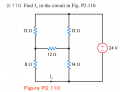I was able to find the equivalent resistance and source current (I = 2.14 A), but the problem arises when I try to keep track of which wire Io is flowing through as I simplify the circuit.

First I wye-delta transformed the 8,12,12 ohm resistor connection on the left and simplified the circuit to something like this: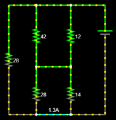Now apparently the current in the blue wire corresponds to Io from the problem, and I can kind of see that since in the original, Io is between the 8 and 14 ohm resistor connection, and here Io is also between the same two resistor connections (albeit the 8 ohm resistor turns into a 28 ohm one from the transformation).

However when I further simplify the circuit to this: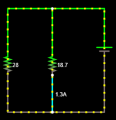Apparently this blue wire also corresponds to the wire Io flows through. I don't understand why that's the case; intuitively for me at least, the current in this particular wire should be completely different than the current in the blue wire in the second image. In the second image, it looks like the addition of the 1.3A current and the current from the 14 ohm resistor should equal the source current, yet the third image implies that the 1.3A current and the far left 28 ohm current equals the source current.

Is there some sort of trick to tell which wire carries the current Io throughout simplification?

(Also as an aside, is there any reason why I can't apply current division to the 28 and 14 ohm parallel resistors in the second image? Is it because the current is also being split to the far-left 28 ohm resistor?)

#### WBahn

Joined Mar 31, 2012
26,398
The surest way to do it is to put a current-sense resistor in the branch you want to know the current for and set it to 0 Ω. Then as you make transformations, you need to be sure that you keep that resistor intact. If you do something like a delta-wye transform involving it, you lose touch with the current you want. That's fine, as long as you know it happened. You can continue to solve for the voltages and currents and then go back to an earlier version of the circuit that still had the current-sense resistor in place and solve for the current through it.

BTW, this is also a very common technique by which to measure the current through a node in a simulation -- with another common way being basically the same thing, namely putting a 0 V voltage source in the path of interest.

#### MrAl

Joined Jun 17, 2014
8,479
Hi, I'm pretty new to circuits and I'm trying to solve the following textbook problem:

View attachment 218699
I was able to find the equivalent resistance and source current (I = 2.14 A), but the problem arises when I try to keep track of which wire Io is flowing through as I simplify the circuit.

First I wye-delta transformed the 8,12,12 ohm resistor connection on the left and simplified the circuit to something like this:
View attachment 218701
Now apparently the current in the blue wire corresponds to Io from the problem, and I can kind of see that since in the original, Io is between the 8 and 14 ohm resistor connection, and here Io is also between the same two resistor connections (albeit the 8 ohm resistor turns into a 28 ohm one from the transformation).

However when I further simplify the circuit to this:
View attachment 218704

Apparently this blue wire also corresponds to the wire Io flows through. I don't understand why that's the case; intuitively for me at least, the current in this particular wire should be completely different than the current in the blue wire in the second image. In the second image, it looks like the addition of the 1.3A current and the current from the 14 ohm resistor should equal the source current, yet the third image implies that the 1.3A current and the far left 28 ohm current equals the source current.

Is there some sort of trick to tell which wire carries the current Io throughout simplification?

(Also as an aside, is there any reason why I can't apply current division to the 28 and 14 ohm parallel resistors in the second image? Is it because the current is also being split to the far-left 28 ohm resistor?)
It looks like the currents you had calculated are close but i am not sure how accurate you want to calculate the solutions.

So are you still having a problem with this?

#### Syph

Joined Oct 3, 2020
4
The surest way to do it is to put a current-sense resistor in the branch you want to know the current for and set it to 0 Ω. Then as you make transformations, you need to be sure that you keep that resistor intact. If you do something like a delta-wye transform involving it, you lose touch with the current you want. That's fine, as long as you know it happened. You can continue to solve for the voltages and currents and then go back to an earlier version of the circuit that still had the current-sense resistor in place and solve for the current through it.

BTW, this is also a very common technique by which to measure the current through a node in a simulation -- with another common way being basically the same thing, namely putting a 0 V voltage source in the path of interest.
If I'm interpreting this correctly, you're recommending that I put a 0 Ω resistor along the wire that contains Io from the problem, and keeping track of that resistor through simplification?

If I do that, I can definitely see where Io is located after the delta-wye transformation. But I'm still having trouble figuring out why that 0 Ω resistor would not disappear after I collapse the parallel circuit in the second image.

(Pretend the 10 mΩ resistor is the 0 Ω resistor here as I can't put a 0 Ω resistor in this simulation)
I don't see how that current-sense resistor goes from here, at the bottom node: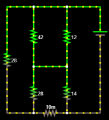To here, in the middle wire: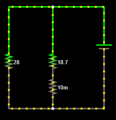After I collapse the series/parallel connections. Intuitively I feel like the current-sense resistor would no longer remain intact after that simplification.

It looks like the currents you had calculated are close but i am not sure how accurate you want to calculate the solutions.

So are you still having a problem with this?
Do you mean the source current I = 2.14 A or Io = 1.3 A? Both of those matches the textbook solutions so I'm not too worried about the calculations there; I'm just trying to figure out why the Io in the textbook problem, which is located in the bottom left wire, corresponds to the same current as the one passing through the 10 mΩ resistor in my second image.

#### WBahn

Joined Mar 31, 2012
26,398
While you can combine the upper 42 Ω and 12 Ω resistors because they are in parallel, you CANNOT just combine the lower 28 Ω and 14 Ω resistors because they are no longer in parallel -- your current sense resistor prevents that.

Also, ask if your simplification makes sense. Before your did it, it was obvious that whatever current flows in the left hand 28 Ω resistor must flow through the current sense resistor to get back to the supply. But afterward you can see that NONE of it does. Clearly something is wrong.

#### MrAl

Joined Jun 17, 2014
8,479
Do you mean the source current I = 2.14 A or Io = 1.3 A? Both of those matches the textbook solutions so I'm not too worried about the calculations there; I'm just trying to figure out why the Io in the textbook problem, which is located in the bottom left wire, corresponds to the same current as the one passing through the 10 mΩ resistor in my second image.
Both. However the answer to your question is easy. 10mOhm compared to the smallest resistor of 8 ohms is 800 times smaller therefore there wont be much difference therefore you will need to use higher precision in your calculation to see any difference.
The 10mOhm resistor obviously changes the total resistance of the 8 Ohm branch making it 8.01 Ohms so the difference will be small. If you use higher precision though, like 2.14 and 1.29 then you may see the difference, although you may have to go to even higher precision than that such sa 6 significant digits for each. Then you will definitely see a difference.

So go back over it and calculate the results to higher precision and then compare. The difference will be small and possibly very hard to measure in real life but in the pure math calculations it will show up for sure simply because you changed the circuit values somewhat.

If by chance you do not see a difference using six digits or more then you did the calculations wrong#### WBahn

Joined Mar 31, 2012
26,398
If I'm interpreting this correctly, you're recommending that I put a 0 Ω resistor along the wire that contains Io from the problem, and keeping track of that resistor through simplification?

If I do that, I can definitely see where Io is located after the delta-wye transformation. But I'm still having trouble figuring out why that 0 Ω resistor would not disappear after I collapse the parallel circuit in the second image.

(Pretend the 10 mΩ resistor is the 0 Ω resistor here as I can't put a 0 Ω resistor in this simulation)
I don't see how that current-sense resistor goes from here, at the bottom node:
View attachment 218771
To here, in the middle wire:
View attachment 218769
After I collapse the series/parallel connections. Intuitively I feel like the current-sense resistor would no longer remain intact after that simplification.

Do you mean the source current I = 2.14 A or Io = 1.3 A? Both of those matches the textbook solutions so I'm not too worried about the calculations there; I'm just trying to figure out why the Io in the textbook problem, which is located in the bottom left wire, corresponds to the same current as the one passing through the 10 mΩ resistor in my second image.
They are NOT the same current -- they are two different currents that just happen to have the same numerical value in this particular problem.

You are seeing an artifact of the specific values in this case. In the first circuit above, the current in the sense resistor is clearly the sum of the currents in the two 28 Ω resistors, while (if you give it a bit of thought), the current in the sense resistor in the second circuit is the sum of the currents in the 28 Ω and 14 Ω resistors that are connected to the bridge in the middle. Hence the only way that the two currents can be the same is if the current in the far left 28 Ω resistor happens to be exactly equal to the current in the 14 Ω resistor. Certainly there is nothing dictating that this is the case, since I could change the 14 Ω resistor to whatever I wanted without affecting the far left 28 Ω resistance that comes about as a result of the delta-wye transformation you did and doing so would affect the current in it but have no effect on the current in that 28 Ω resistor. So if they do happen to be equal in this circuit, it is by coincidence. Well, it turns out that, by coincidence, they are both equal to 6/7 A (0.857 A).

#### Syph

Joined Oct 3, 2020
4
Thanks, I also had a feeling it might have been a coincidence.
I managed to solve the problem by doing a delta to wye transform instead of a wye to delta (with the three 12 ohm resistors). This way was a lot easier to keep track of the current, especially with your suggestion of using a current-sense resistor.

#### WBahn

Joined Mar 31, 2012
26,398
I would have used mesh analysis because then one of the unknowns is the current I am interested in. Have you learned mesh analysis yet?

#### Syph

Joined Oct 3, 2020
4
...while (if you give it a bit of thought), the current in the sense resistor in the second circuit is the sum of the currents in the 28 Ω and 14 Ω resistors that are connected to the bridge in the middle.
Sorry to pry further, but I just wanted to check if my logic as to why this is is correct.

I'm assuming that by applying KCL onto the first circuit, I see that the current in the far-left 28 Ω resistor plus the sum of the currents from the other 28 Ω and 14 Ω resistor should equal to the current from the voltage source.

In the second circuit, we see that by KCL, the current from the far-left 28 Ω resistor plus the current from the final simplified 18.7 Ω resistor equals the source current.

Extrapolating from that, we find that the sum of the currents of the 28 and 14 Ω resistor must equal to the current from the 18.7 Ω resistor (or the current in the sense resistor).

Is that correct, or is there an easier proof for that?

I would have used mesh analysis because then one of the unknowns is the current I am interested in. Have you learned mesh analysis yet?
Yes I did learn that. However, my instructor said that this problem was solvable using only delta-wye and current/voltage division, so I wanted to stick with those methods as sometimes in an exam we are not given the freedom to use any method we want.

#### WBahn

Joined Mar 31, 2012
26,398

You definitely have to use what they tell you to. Hopefully once they have tested you on the specific techniques they will let you decide which approach to use for a given problem.

#### BobaMosfet

Joined Jul 1, 2009
1,850
Hi, I'm pretty new to circuits and I'm trying to solve the following textbook problem:

View attachment 218699
I was able to find the equivalent resistance and source current (I = 2.14 A), but the problem arises when I try to keep track of which wire Io is flowing through as I simplify the circuit.

First I wye-delta transformed the 8,12,12 ohm resistor connection on the left and simplified the circuit to something like this:
View attachment 218701
Now apparently the current in the blue wire corresponds to Io from the problem, and I can kind of see that since in the original, Io is between the 8 and 14 ohm resistor connection, and here Io is also between the same two resistor connections (albeit the 8 ohm resistor turns into a 28 ohm one from the transformation).

However when I further simplify the circuit to this:
View attachment 218704

Apparently this blue wire also corresponds to the wire Io flows through. I don't understand why that's the case; intuitively for me at least, the current in this particular wire should be completely different than the current in the blue wire in the second image. In the second image, it looks like the addition of the 1.3A current and the current from the 14 ohm resistor should equal the source current, yet the third image implies that the 1.3A current and the far left 28 ohm current equals the source current.

Is there some sort of trick to tell which wire carries the current Io throughout simplification?

(Also as an aside, is there any reason why I can't apply current division to the 28 and 14 ohm parallel resistors in the second image? Is it because the current is also being split to the far-left 28 ohm resistor?)
Title: Understanding Basic Electronics, 1st Ed.
Publisher: The American Radio Relay League
ISBN: 0-87259-398-3